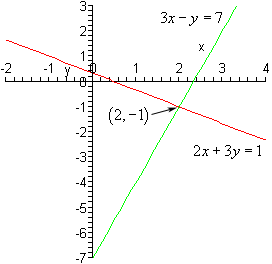# AP Board Class 9 Maths Chapter 6 Linear Equations in Two Variables

By the time students reach Class 9, they are already familiar with linear equations and problems based on this concept. However, in earlier class students are basically taught how to solve linear equations with one variable. In Class 9, students are introduced to the concept of linear equations in two variables. They are also taught about several methods to solve linear equation, either algebraically or graphically.

## Linear Equations In Two Variable

A linear equation having two variables is termed as a linear equation in two variables. Any pair of values of ‘a’ and ‘b,’ which satisfies the linear equation in two variables is said to be its solution. However, a linear equation in two variables has many solutions.

### Graph Of A Linear Equation In Two Variables

Here, what students need to understand is that the graphical representation of a linear equation in two variables is a straight line.

### How To Draw The Graph Of A Linear Equation

• Write down the linear equation.
• Use x = 0 in the given equation to find the corresponding value of y.
• Similarly, use y = 0 in the given equation to find the corresponding value of x.
• Then, jot down the values of x and y as coordinates of x and y respectively as (x, y) form.
• Use the numbers to plot the points on the graph.
• Finally, join these points. So, whatever line is drawn in the graph is the linear equations in two variables.

Notably, it is always better to take more than two points. To check the accuracy of the answer.

Students can also refer to some of the chapter questions along with their solutions below to gain a clearer idea of the concept.

Question 1: Express 3x – 7 = 0 as ax + by + c = 0 and mention the values of a, b and c.

Solution: 3x – 7 = 0 can be written as 3x – 0.

y – 7 = 0.

Therefore: a = 3, b = 0 and c = -7.

Question 2: A number is 27 more than the number obtained by reversing its digits. If its unit’s and ten’s digits are x and y respectively, write the linear equation representing the above statement.

Solution: Given that units digit is represented by x and tens digit by y, then the number is 10y + x. Now reverse the digits, then the new number would be 10x + y (Recall the place value of digits in a two-digit number).
Hence, as per the given condition the equation is (two-digit number) – (number formed by reversing the digits) = 27.
That is, 10y + x – (10x + y) = 27
10y + x – 10x -y – 27 = 0
So, 9y – 9x – 27 = 0
and y – x – 3 = 0
Hence, x -y + 3 = 0 is the required equation.

Question 3: Find the solution for 3x-y = 7 and 2x+3y = 1

Solution: You need to plot the given equation on the graphs and then you will get a point of intersection. Take the number from this point and this is basically the solution to the equations which is (2, -1).Get the latest information about AP Board and access various study materials, including syllabus, timetable, hall tickets only at BYJU’s.